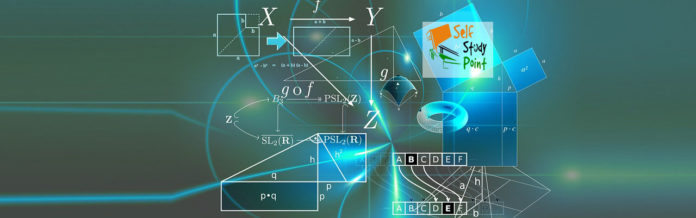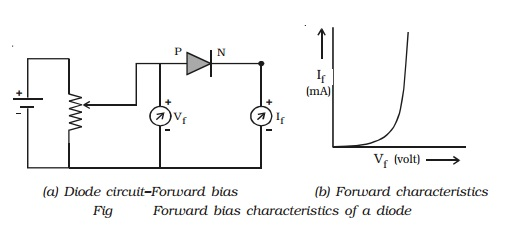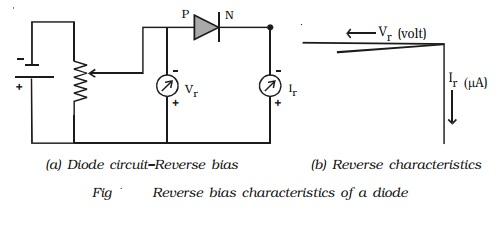### Forwarding Bias Characteristics of PN Junction Diode

The circuit for the study of forwarding bias characteristics of PN junction diode is shown in Fig a. The voltage between P-end and N-end is increased from zero in suitable equal steps and the corresponding currents are noted down. Fig b shows the forward bias characteristic curve of the diode. Voltage is the independent variable. Therefore, it is plotted along X-axis. Since the current is the dependent variable, it is plotted against Y-axis.From the characteristic curve, the following conclusions can be made:

1. The forward characteristic is not a straight line. Hence the ratio V/I is not a constant (i.e.) the diode does not obey Ohm’s law. This implies that the semiconductor diode is a non-linear conductor of electricity.
2. It can be seen from the characteristic curve that initially, the current is very small.

### Reverse bias characteristics:

The circuit for the study of reverse bias characteristics of PN junction diode is shown in Fig a. The voltage is increased from zero in suitable steps. For each voltage, the corresponding current readings are noted down. Fig b shows the reverse bias characteristic curve of the diode. From the characteristic curve, it can be concluded that, as the voltage is increased from zero, reverse current (in the order of microamperes) increases and reaches the maximum value at a small value of the reverse voltage.When the voltage is further increased, the current is almost independent of the reverse voltage up to a certain critical value. This reverse current is known as the reverse saturation current or leakage current.